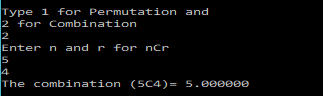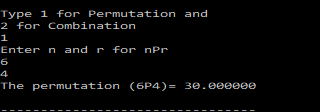# Permutation and Combination – C PROGRAM

In one of my posts on C Programming, I showed, how to calculate the Factorial of a number.

In this post we will use that program, and extend it to calculate the Combination$^nC_r$ and the Permutation$^nP_r$.

If you haven’t read the post on factorial calculation, then you can check it out here.

Now, a combination is defined as:$^nC_r = \frac{n!}{r!(n-r)!}$

and the Permutation is defined as:$^nP_r = \frac{n!}{(n-r)!}$

So we will have the user enter their choice, and then prompt the user to enter the$r$ and$n$
and then return the answer based on their choice.

The program is pretty simple and can be written as shown below.

### PROGRAM:

/************************************
****PERMUTATION AND COMBINATION*****
***********************************/
#include<stdio.h>

/*Function that calculates the factorial of a given integer n */
double factorial(int n){
int i;
double fact=1;
for(i=n;i>=1;i--){
fact=fact*i;
}
return fact;
}

int main(){
int n,r,choice;
printf("\n\nType 1 for Permutation and \n2 for Combination\n");
scanf("%d",&choice);
if(choice==1){
printf("Enter n and r for nPr\n");
scanf("%d %d",&n,&r);
if(r>n){
printf("Sorry! r should be smaller than n\n");
return 0;
}
printf("The permutation (%dP%d)= %f\n",n,r,factorial(n)/factorial(n-r));
}else if(choice==2){
printf("Enter n and r for nCr\n");
scanf("%d %d",&n,&r);
if(r>n){
printf("r should be smaller than n\n");
return 0;
}
printf("The combination (%dC%d)= %f\n",n,r,factorial(n)/(factorial(n-r)*factorial(r)));
}
else{
printf("\nPlease enter a valid choice!\n");
}
}


As one can see, the program is pretty much self-explanatory.

### OUTPUT:

Some sample outputs have been attached below:[wpedon id="7041" align="center"]

## 5 thoughts on “Permutation and Combination – C PROGRAM”

1. Permutation formula is wrong

1. Thanks a lot for the correction!

1. BTW thank you so much. Your programs mean a lot!!!

2. Permutation
n!/(n-r)!

1. Updated